Publicité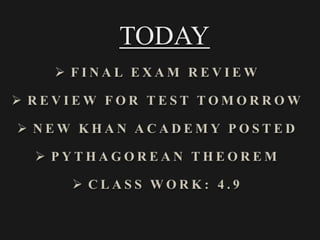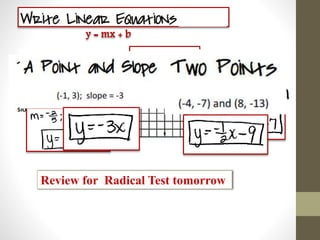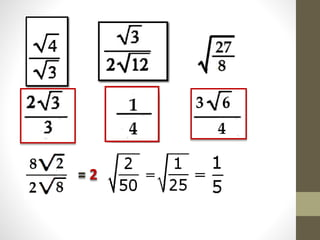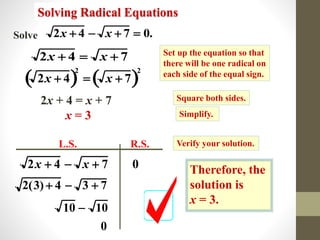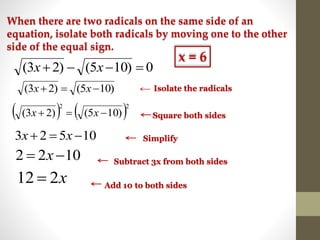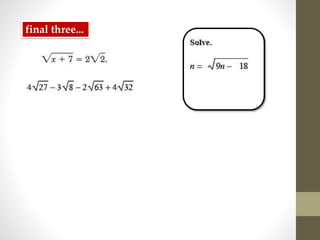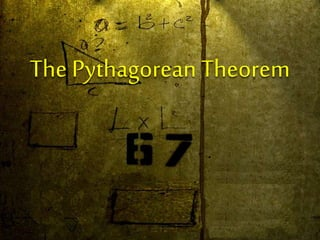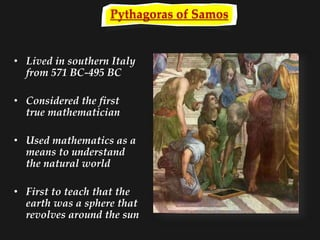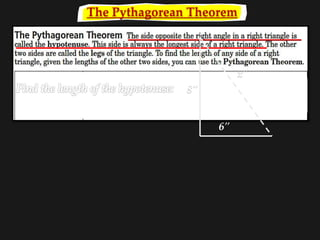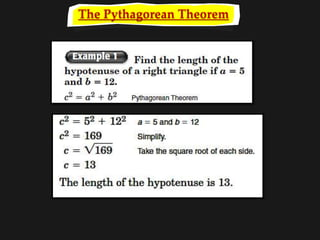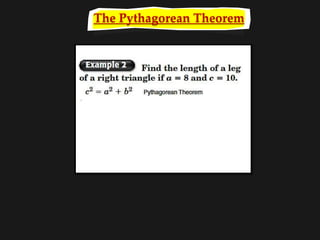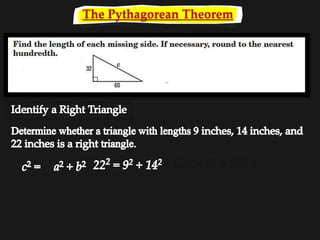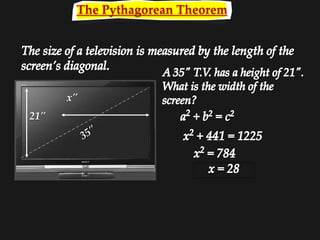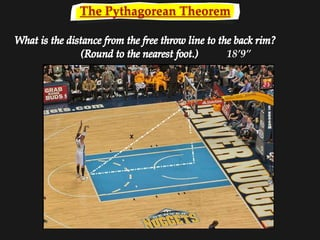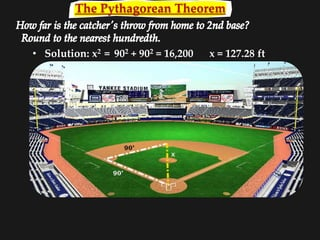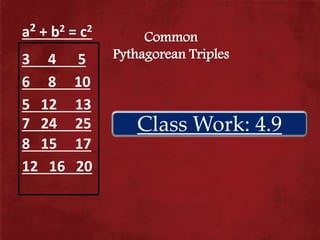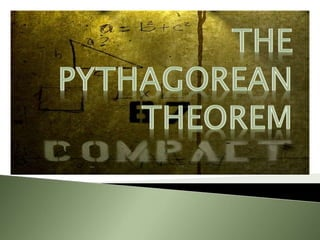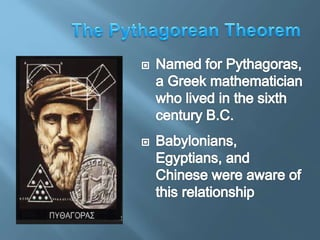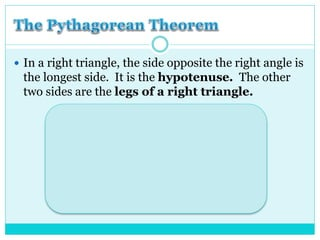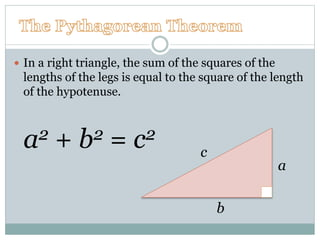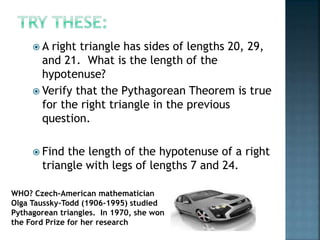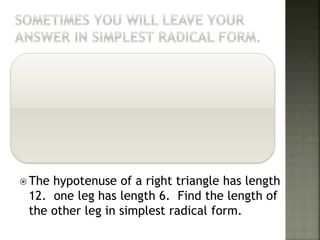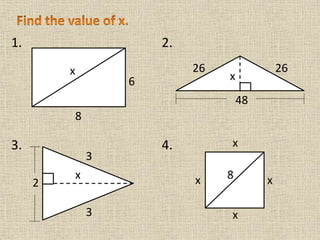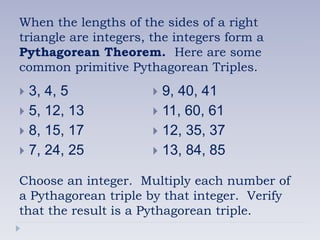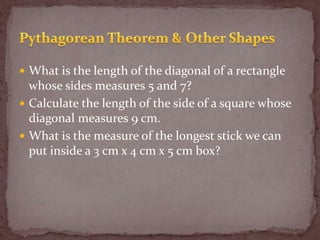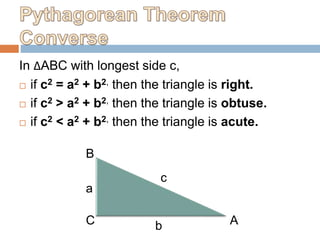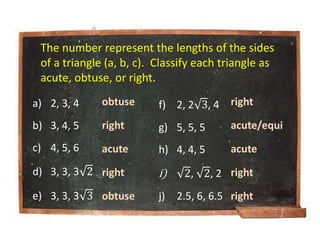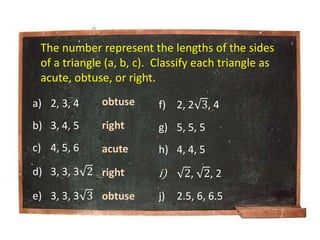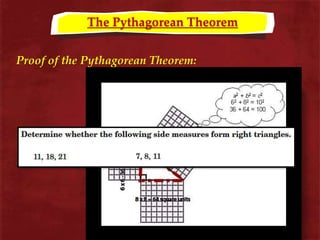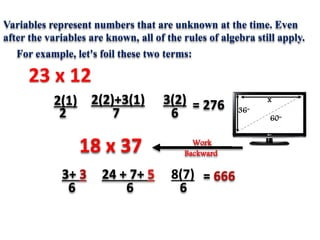1 sur 31
Publicité

### May 11, 2015

1. TODAY
2. y = mx + b Review for Radical Test tomorrow
3. = 2
4. 2x  4  x  7  0. Set up the equation so that there will be one radical on each side of the equal sign. 2x  4  x  7 Square both sides. 2x  4  2  x  7  2 Simplify. 2x + 4 = x + 7 x = 3 Verify your solution. 2x  4  x  7 0 Therefore, the solution is x = 3. Solve Solving Radical Equations 2(3)  4  3  7 10  10 0 L.S. R.S.
5. 0)105()23(  xx )105()23(  xx    22 )105()23(  xx 10523  xx 1022  x x212  Isolate the radicals Square both sides Simplify Subtract 3x from both sides Add 10 to both sides When there are two radicals on the same side of an equation, isolate both radicals by moving one to the other side of the equal sign. x = 6
6. final three...
7. The Pythagorean Theorem
8. Pythagoras of Samos • Lived in southern Italy from 571 BC-495 BC • Considered the first true mathematician • Used mathematics as a means to understand the natural world • First to teach that the earth was a sphere that revolves around the sun
9. The Pythagorean Theorem 8" 6" x"
10. The Pythagorean Theorem
11. The Pythagorean Theorem
12. The Pythagorean Theorem 484 = 81 + 196 ?
13. The Pythagorean Theorem 21" x"
14. The Pythagorean Theorem 18’9”
15. The Pythagorean Theorem • Solution: x2 = 902 + 902 = 16,200 x = 127.28 ft
16. Class Work: 4.9 Common Pythagorean Triples 6 8 10 5 12 13 7 24 25 8 15 17 3 4 5 a2 + b2 = c2 12 16 20
17.  In a right triangle, the side opposite the right angle is the longest side. It is the hypotenuse. The other two sides are the legs of a right triangle. legs hypotenuse
18.  In a right triangle, the sum of the squares of the lengths of the legs is equal to the square of the length of the hypotenuse. a2 + b2 = c2 a b c
19.  A right triangle has sides of lengths 20, 29, and 21. What is the length of the hypotenuse?  Verify that the Pythagorean Theorem is true for the right triangle in the previous question.  Find the length of the hypotenuse of a right triangle with legs of lengths 7 and 24. WHO? Czech-American mathematician Olga Taussky-Todd (1906-1995) studied Pythagorean triangles. In 1970, she won the Ford Prize for her research
20.  Find the value of x. Leave your answer in simplest radical form.  The hypotenuse of a right triangle has length 12. one leg has length 6. Find the length of the other leg in simplest radical form. 8 20 x
21. 1. 2. 3. 4. x 8 6 x x x x8 x 26 26 48 2 x 3 3
22. When the lengths of the sides of a right triangle are integers, the integers form a Pythagorean Theorem. Here are some common primitive Pythagorean Triples.  3, 4, 5  5, 12, 13  8, 15, 17  7, 24, 25 Choose an integer. Multiply each number of a Pythagorean triple by that integer. Verify that the result is a Pythagorean triple.  9, 40, 41  11, 60, 61  12, 35, 37  13, 84, 85
23.  What is the length of the diagonal of a rectangle whose sides measures 5 and 7?  Calculate the length of the side of a square whose diagonal measures 9 cm.  What is the measure of the longest stick we can put inside a 3 cm x 4 cm x 5 cm box?
24. In ΔABC with longest side c,  if c2 = a2 + b2, then the triangle is right.  if c2 > a2 + b2, then the triangle is obtuse.  if c2 < a2 + b2, then the triangle is acute. B C A a b c
25. a) 2, 3, 4 b) 3, 4, 5 c) 4, 5, 6 d) 3, 3, 3 2 e) 3, 3, 3 3 f) 2, 2 3, 4 g) 5, 5, 5 h) 4, 4, 5 i) 2, 2, 2 j) 2.5, 6, 6.5 The number represent the lengths of the sides of a triangle (a, b, c). Classify each triangle as acute, obtuse, or right. obtuse right acute right obtuse right acute/equi acute right right
26. a) 2, 3, 4 b) 3, 4, 5 c) 4, 5, 6 d) 3, 3, 3 2 e) 3, 3, 3 3 f) 2, 2 3, 4 g) 5, 5, 5 h) 4, 4, 5 i) 2, 2, 2 j) 2.5, 6, 6.5 The number represent the lengths of the sides of a triangle (a, b, c). Classify each triangle as acute, obtuse, or right. obtuse right acute right obtuse
27. The Pythagorean Theorem Proof of the Pythagorean Theorem:
28. 23 x 12 3(2)2(2)+3(1)2(1) 672 = 276 18 x 37 8(7) 6 24 + 7+ 5 6 3+ 3 6 = 666 Work Backward Variables represent numbers that are unknown at the time. Even after the variables are known, all of the rules of algebra still apply. For example, let's foil these two terms: 60" 36" x
Publicité